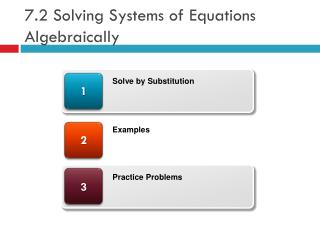DownloadDownload Presentation7.2 Solving Systems of Equations Algebraically

# 7.2 Solving Systems of Equations Algebraically

Télécharger la présentation## 7.2 Solving Systems of Equations Algebraically

- - - - - - - - - - - - - - - - - - - - - - - - - - - E N D - - - - - - - - - - - - - - - - - - - - - - - - - - -
##### Presentation Transcript

1. 7.2 Solving Systems of Equations Algebraically 1 Solve by Substitution 2 Examples 3 Practice Problems

2. Definitions • System of Equations • 2 or more equations on the same coordinate system • Consistent System • Graph of equations cross each other (not parallel) • System has a solution • Slope is different • Inconsistent System • Graph of equations do not cross each other (parallel) • System does not have a solution • Slope is the same

3. Consistent System • Has a solution at (1,2) (1,2)

4. Inconsistent System • No Solution

5. Solve by Substitution • Steps • Solve for x in the first equation • Substitute the answer in for x in the second equation • Solve for y • Substitute the value of y into either of the original equations • Solve for x • Take the value of x and y and put them in ordered pair form (x,y)

6. Solve by Substitution Example

7. Solve by Substitution Example (Cont.)

8. Practice Problems • Page 379 Problems 11-27